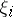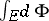# Kolmogorov integral

(diff) ← Older revision | Latest revision (diff) | Newer revision → (diff)
A general scheme for constructing an integral, including the Lebesgue–Stieltjes integral, the Burkill integral, the Hellinger integral, etc. Introduced by A.N. Kolmogorov . One considers a directed family of partitions of a spaceof arbitrary nature. A set function(generally many-valued) is defined on the elements of the partition. The sum of the values of this function taken over all elements of the partition gives a many-valued function of the partition. In particular, this sum is a generalization of the Riemann sumwhere the multi-valuedness is a consequence of the arbitrariness in the choice of the pointson the elements of the partition. The directed limit of the partition functions then defines the Kolmogorov integral. The Kolmogorov integral is considered both for finite and countable partitions. The Kolmogorov integral can be considered for functions with values in a commutative topological group.